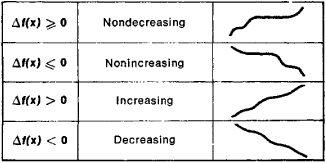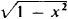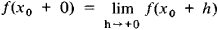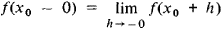# Monotonic Function

(redirected from Monotone increasing)

## monotonic function

[¦män·ə¦tän·ik ′fəŋk·shən]
(mathematics)

## Monotonic Function

(or monotone function), a function whose increments Δf(x) = f(x′) − f(x) do not change sign when Δx = x′ − x > 0; that is, the increments are either always nonnegative or always nonpositive. Somewhat inaccurately, a monotonic function can be defined as a function that always varies in the same direction. Different types of monotonic functions are represented in Figure 1. For example, the function y = x3 is an increasing function. If a function f(x) has a derivative f′(x) that is nonnegative at every point and that vanishes only at a finite number of individual points, then f(x) is an increasing function. Similarly, if f′(x) ≤ 0 and vanishes only at a finite number of points, then f(x) is a decreasing function.Figure 1

A monotonicity condition can hold either for all x or for x on a given interval. In the latter case, the function is said to be monotonic on this interval. For example, the function y =increases on the interval [−1,0] and decreases on the interval [0, +1]. A monotonic function is one of the simplest classes of functions and is continually encountered in mathematical analysis and the theory of functions. If f(x) is a monotonic function, then the following limits exist for any X0:andReferences in periodicals archive ?
([H.sub.0]) g(0, y) = 0, for ally [greater than or equal to] 0; ([partial derivative]g/[partial derivative]x)(x, y) [greater than or equal to] 0 (or g(x, y) is a strictly monotone increasing function with respect to x when f [equivalent to] 0) and ([partial derivative]g/[partial derivative]y)(x, y) [less than or equal to] 0, for all x [greater than or equal to] 0 and y [greater than or equal to] 0.
Here the sequence {[u.sub.n]} is monotone increasing and the order interval [[u.sub.m], [right arrow]) is closed and convex.
Particularly, the reflected phase will turn to monotone increasing near resonance frequency due to the pure imaginary characteristic of graphene's conductivity, thus providing conditions for positive reflected group delay.
In Theorem 3, suppose that the function [rho](t) is monotone increasing; then the sequence [[R.sub.n]] has only positive members and the assumption
We strictly define t as the monotone increasing function of both [n.sub.j] and [s.sub.j].
Proposition 3.1 is not implied in Walter's comparison theorem for monotone increasing kernels [20, II.
and by Theorem 1 we know that any bounded, monotone increasing solution must satisfy [beta]'(0) = 0, 0 < [beta](0) < 36V, and [beta](1) = 1.5.
For 0 < d < 1, l > 0 the function [r.sub.d,l](s) is monotone increasing in [MATHEMATICAL EXPRESSION NOT REPRODUCIBLE IN ASCII]
where the continuous function a : [R.sub.+] [right arrow] [R.sub.+] is strictly monotone increasing with a(0) = 0 and a(t) [right arrow] [infinity] as t [right arrow] [infinity].
It is well known that [x.sub.n] = [(1 + (1/n)).sup.n] and [y.sub.n] = [(1 + (1/n)).sup.n+1] are, respectively, monotone increasing and monotone decreasing, and both of them converge to the constant e.
(i) When r < [r.sub.1], (2) has a monotone decreasing kink profile solitary wave solution U([xi]) satisfying U(-[infinity]) = [p root of [[phi].sub.1]], U(+[infinity]) = 0, and a monotone increasing kink profile solitary wave solution U([xi]) satisfying U(-[infinity]) = -[p root of [[phi].sub.1]], U(+[infinity]) = 0.
[MATHEMATICAL EXPRESSION NOT REPRODUCIBLE IN ASCII] For a given exponent [alpha] [member of] (0,1/2), there exists a monotone increasing function l(*) such that

Site: Follow: Share:
Open / Close